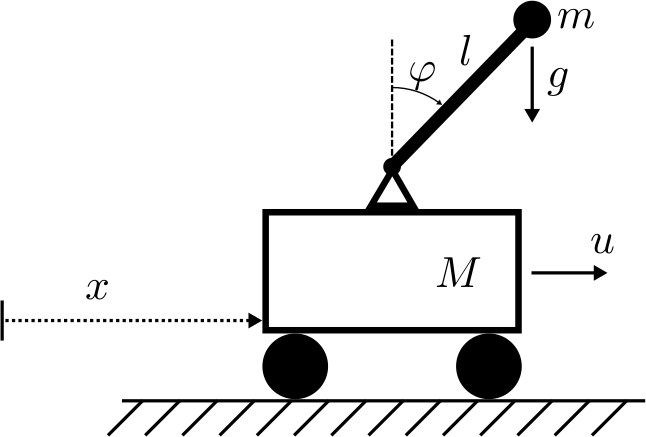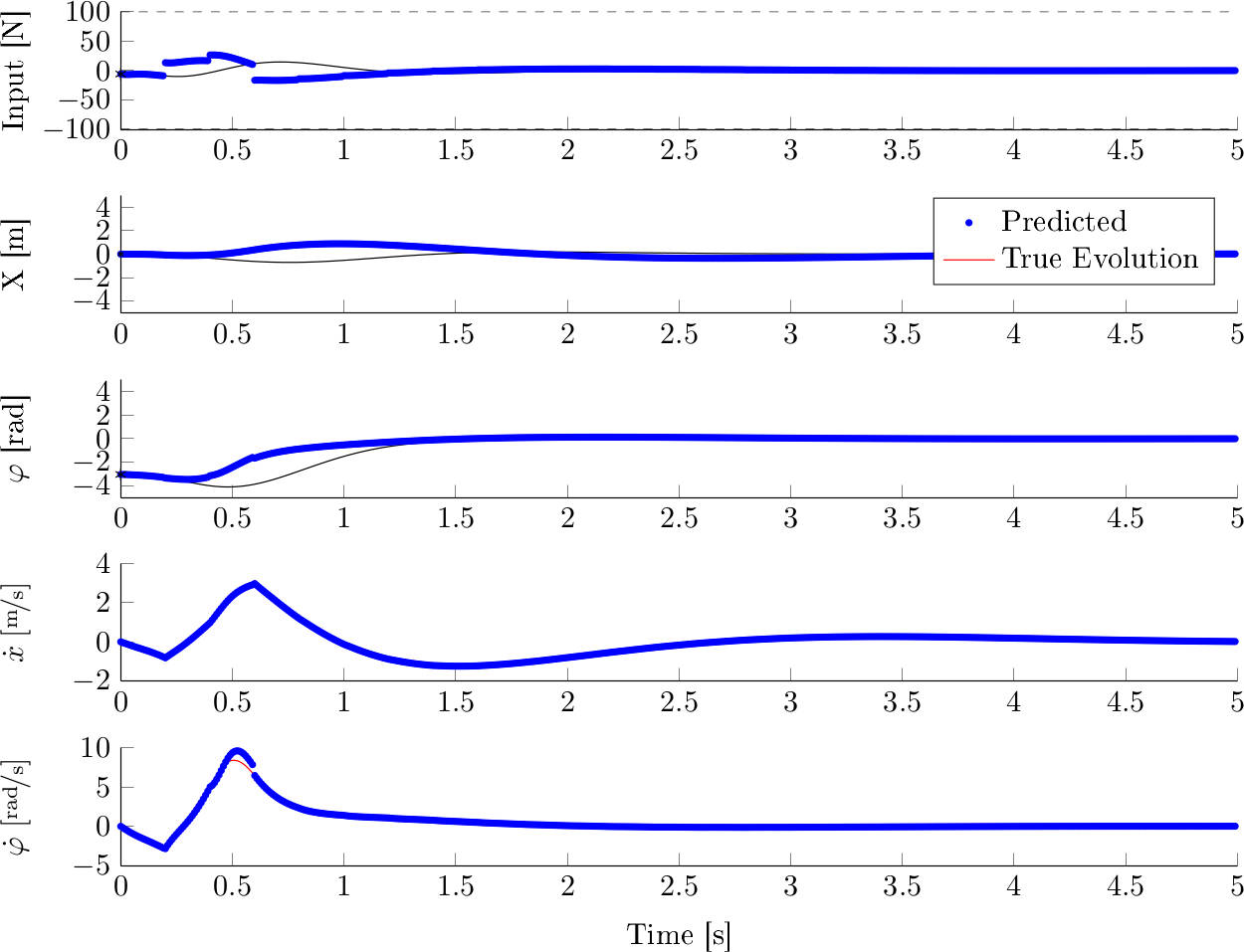My semester thesis titled Nonlinear Infinite Horizon Model Predictive Control with Parametric Trajectories explores a new way to parametrize trajectories for infinite horizon model predictive control.

Here is the abstract:

This work shows how infinite horizon model predictive control (MPC) can be combined with parametric trajectories for control of nonlinear systems. We use exponentially decaying basis functions to approximate state and input trajectories in time. A variational formulation allows trajectory cost and constraints of the optimization problem to be expressed in terms of parameters describing the trajectories. Optimization is done over those parameters via sequential quadratic programming. A full MATLAB implementation of this MPC routine is developed. Results are tested in simulation on an inverted pendulum on a cart. We show that the controller finds trajectories that enable a swing-up of the pendulum and stabilization around its upper equilibrium. A testing environment with a real system is prepared for further research on this control scheme.